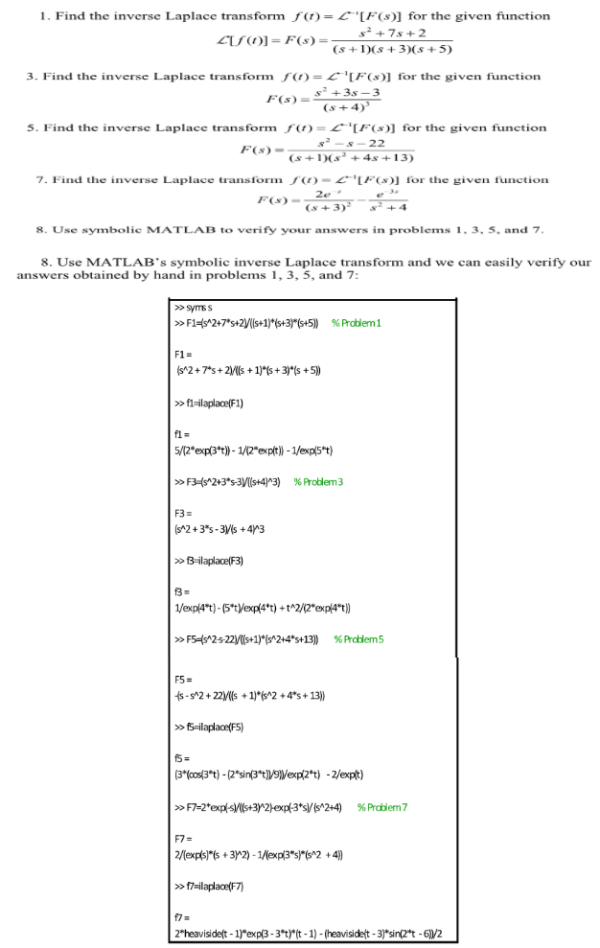Use MATLAB's symbolic inverse Laplace transform and we can easily verify our answers obtained by hand in problems 1, 3, 5, and 7.Use MATLAB's symbolic inverse Laplace transform and we can easily verify our answers obtained by hand in problems 1, 3, 5, and 7.

System Dynamics Page 2 dynamics dynamics dynamics dynamics dynamics dynamics dynamics System dynamics Page 3